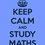# Help needed!

If $a, b, c, d\in\mathbb{R}$ such that

$|a-b|+|c-d|=99$

$|a-c|+|b-d|=1$

then the possible value(s) of $|a-d|+|b-c|$ are.....Note by Aneesh Kundu
6 years, 6 months ago

This discussion board is a place to discuss our Daily Challenges and the math and science related to those challenges. Explanations are more than just a solution — they should explain the steps and thinking strategies that you used to obtain the solution. Comments should further the discussion of math and science.

When posting on Brilliant:

• Use the emojis to react to an explanation, whether you're congratulating a job well done , or just really confused .
• Ask specific questions about the challenge or the steps in somebody's explanation. Well-posed questions can add a lot to the discussion, but posting "I don't understand!" doesn't help anyone.
• Try to contribute something new to the discussion, whether it is an extension, generalization or other idea related to the challenge.

MarkdownAppears as
*italics* or _italics_ italics
**bold** or __bold__ bold
- bulleted- list
• bulleted
• list
1. numbered2. list
1. numbered
2. list
Note: you must add a full line of space before and after lists for them to show up correctly
paragraph 1paragraph 2

paragraph 1

paragraph 2

[example link](https://brilliant.org)example link
> This is a quote
This is a quote
    # I indented these lines
# 4 spaces, and now they show
# up as a code block.

print "hello world"
# I indented these lines
# 4 spaces, and now they show
# up as a code block.

print "hello world"
MathAppears as
Remember to wrap math in $$ ... $$ or $ ... $ to ensure proper formatting.
2 \times 3 $2 \times 3$
2^{34} $2^{34}$
a_{i-1} $a_{i-1}$
\frac{2}{3} $\frac{2}{3}$
\sqrt{2} $\sqrt{2}$
\sum_{i=1}^3 $\sum_{i=1}^3$
\sin \theta $\sin \theta$
\boxed{123} $\boxed{123}$

Sort by:

- 6 years, 6 months ago

- 6 years, 6 months ago

Don't know that.

Can u state an example for each of the values? It would be really helpful.

- 6 years, 6 months ago

consider a>b>c>d, then plug in to open mod. then solve by cases. ans is 1,98,99,100.

- 6 years, 6 months ago

If $a>b>c>d$ then we can remove the modulus from both the equations. Rearranging the last equation we get our expression, but that would give us the value to be 1.

Cases is not really a gud option (24 cases is just tooooo much).

- 6 years, 6 months ago

where did you get the question from . actually you need not solve 24 cases.most of them are same

- 6 years, 6 months ago

Oh... That sounds great I'll try that. My teacher gave me this one.

- 6 years, 6 months ago

I was able to prove that $0\leq |a-d|+|b-c|\leq 100$

I coudn't go any further. I have not verified the equality cases either.

- 6 years, 6 months ago

happy bday

- 6 years, 6 months ago

Thnx a lot. Actually it was yesterday.

- 6 years, 6 months ago